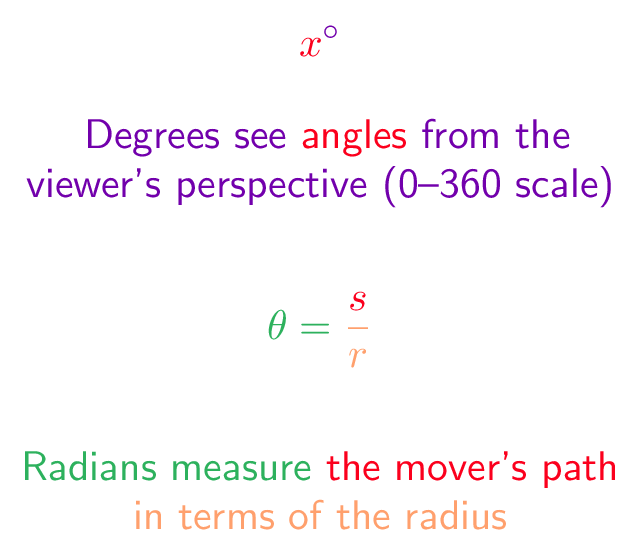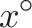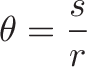## Colorized Definition\newcommand{\degree}{\color{c1}}
\newcommand{\anglecolor}{\color{c3}}
\newcommand{\degreemeasure}{\color{c4}}$\displaystyle{ \degree \anglecolor{x}^{\degree{ \circ }} }$$\displaystyle{ \radian \theta = \anglecolor \radius \frac{\anglecolor s}{r} }$